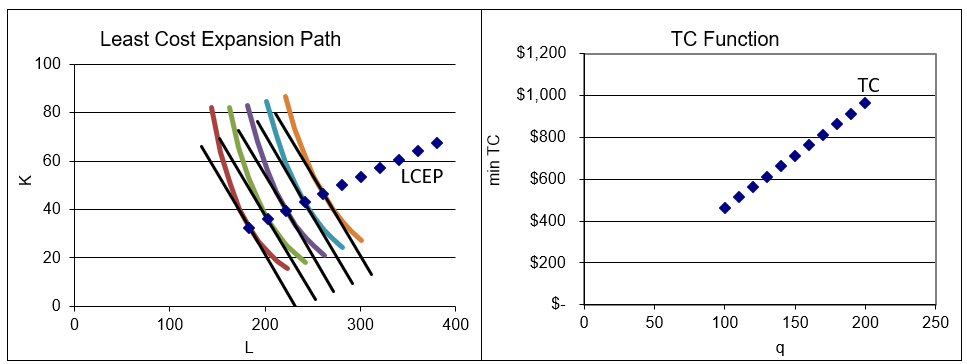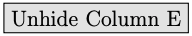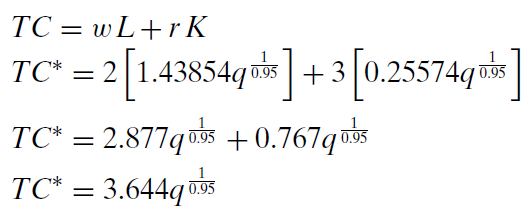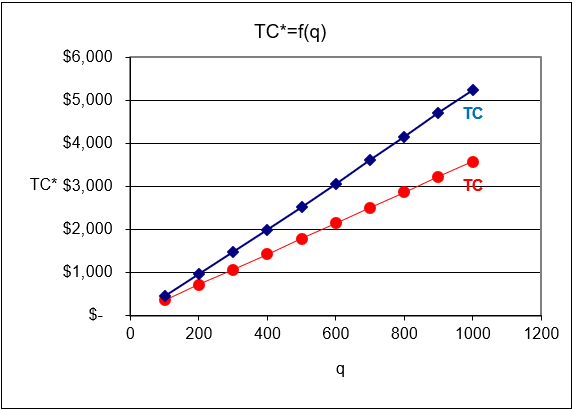# 11.3: Deriving the Cost Function

$$\newcommand{\vecs}{\overset { \rightharpoonup} {\mathbf{#1}} }$$ $$\newcommand{\vecd}{\overset{-\!-\!\rightharpoonup}{\vphantom{a}\smash {#1}}}$$$$\newcommand{\id}{\mathrm{id}}$$ $$\newcommand{\Span}{\mathrm{span}}$$ $$\newcommand{\kernel}{\mathrm{null}\,}$$ $$\newcommand{\range}{\mathrm{range}\,}$$ $$\newcommand{\RealPart}{\mathrm{Re}}$$ $$\newcommand{\ImaginaryPart}{\mathrm{Im}}$$ $$\newcommand{\Argument}{\mathrm{Arg}}$$ $$\newcommand{\norm}{\| #1 \|}$$ $$\newcommand{\inner}{\langle #1, #2 \rangle}$$ $$\newcommand{\Span}{\mathrm{span}}$$ $$\newcommand{\id}{\mathrm{id}}$$ $$\newcommand{\Span}{\mathrm{span}}$$ $$\newcommand{\kernel}{\mathrm{null}\,}$$ $$\newcommand{\range}{\mathrm{range}\,}$$ $$\newcommand{\RealPart}{\mathrm{Re}}$$ $$\newcommand{\ImaginaryPart}{\mathrm{Im}}$$ $$\newcommand{\Argument}{\mathrm{Arg}}$$ $$\newcommand{\norm}{\| #1 \|}$$ $$\newcommand{\inner}{\langle #1, #2 \rangle}$$ $$\newcommand{\Span}{\mathrm{span}}$$

We have solved the input cost minimization problem so the next task is comparative statics analysis. We will focus on shocking q (the quantity the firm must produce) and track minimum total cost. The relationship between $$TC\mbox{*}$$ and q is called the cost function.

The novelty here is that we are not interested in how the optimal values of the endogenous variables, L and K vary as we shock q. Instead, we focus on the objective function, minimum total cost, and how it changes as q changes.

Another important aspect of comparative statics analysis for the input cost minimization problem is that, unlike utility in the Theory of Consumer Behavior, total cost can be cardinally measured. We can compare the total costs of different firms and perform arithmetic on total cost. If the minimum TC for $$q=10$$ is $40 and it rises to$45 when $$q=11$$, we can say TC increased by $5. Because TC is cardinal, we will be able to interpret and use the Lagrangean multiplier. As usual, we will explore both ways to do comparative statics: • Numerical methods using a computer: Excel’s Solver and the Comparative Statics Wizard. • Analytical methods using algebra and calculus: conventional paper and pencil. #### Numerical Methods to Derive the Cost Function STEP Open the Excel workbook DerivingCostFunction.xls, read the Intro sheet, and proceed to the OptimalChoice sheet. The organization is the same as in the InputCostMin.xls workbook. The cost-minimizing way of producing 100 units of output is to use about 183.3 hours of labor with 32.6 machines, which costs$464.38. There is no other combination of L and K that makes 100 units at a lower cost.

What happens if the firm needs to produce more, say, 110 units of output?

STEP Change cell B18 to 110.

The chart updates, showing a new (red) isoquant. The initial combination is not a viable option because it cannot produce 110 units. The firm has to re-optimize.

STEP Run Solver to find the new optimal solution.

The cost-minimizing amounts of labor and capital increase to produce the higher output required and the minimum total cost is now $513.39. We are looking for the minimum total cost. We want to know the cheapest way of producing any given output. This is called the cost function. We can show the comparative statics analysis on the isoquant-isocost graph or on a presentation graph where we plot $$TC\mbox{*}=f(q)$$, ceteris paribus. If we connected the points of tangency of isoquants and isocosts, we would get the least cost expansion path (LCEP). Our work thus far has revealed two points on the LCEP and cost function: when q = 100, TC =$464.38 and when q = 100, TC = $513.39. Let’s use the Comparative Statics Wizard to get more data so we can draw the LCEP and cost functions and understand how they are related. STEP Return cell B18 to 100, then run the Comparative Statics Wizard, applying 10 q shocks in increments of 10. The CS1 sheet shows what your results should look like. The CS1 sheet includes two graphs, the isoquant-isocost graph with the least cost expansion path and the cost function, as shown in Figure 11.10.Figure 11.10: Deriving the cost function. Source: DerivingCostFunction.xls!CS1 Figure 11.10 should remind of you of other graphs we have drawn, such as Engel and demand curves. On the left, using the display of the optimal solution to the input cost minimization problem, we show how different q produce a set of tangency points that comprise the LCEP. On the right in Figure 11.10, we show only the minimum cost of producing each level of q, and hide everything else. This allows us to highlight the relationship between TC and q. The two graphs in Figure 11.10 make clear that the source of the cost function is the optimal solution of the cost minimization problem as q varies. Just like demand curves do not come out of thin air, but are derived from utility maximization, cost functions are derived from input cost minimization. We are interested in the shape of the cost function. It looks like a line, but is it really linear? To find out, we can see if it has a constant slope. If the slope is changing, we know the function is not linear. STEP In your CS sheet, find the slope at different points on the function by computing the change in TC divided by the change in q. Click thebutton (near cell C9 in the CS1 sheet) if you are stuck or to check your work. It is clear that the slope changes as output changes. This means that the cost function is nonlinear. #### Analytical Methods to Derive the Cost Function We can use the Lagrangean method to find $$TC\mbox{*} = f(q)$$. We will leave q as a letter instead of a number so that the reduced-form solution will include q. Then we can plug in any value of q to find minimum cost for that q and easily draw a graph of the cost function. The solution closely follows the work we did at the beginning of this chapter, but we proceed step-by-step to practice and reinforce the Lagrangean method. The problem is $\begin{gathered} %star suppresses line # \min\limits_{L,K}TC=2L+3K\\ \textrm{s.t. } q = L^{0.75}K^{0.2}\end{gathered}$ The first step is to rewrite the constraint so that it is equal to zero. $q - L^{0.75}K^{0.2}=0$ The second step is to form the Lagrangean by adding lambda, $$\lambda$$, times the rewritten constraint to the original objective function. We use an extra-large L for the Lagrangean function that is not at all related to the L for labor. $\begin{gathered} %star suppresses line # \min\limits_{L,K, \lambda}{\large\textit{L}}=2L+3K + \lambda (q- L^{0.75}K^{0.2})\end{gathered}$ The third step to finding the optimal solution is to take the derivative of the Lagrangean with respect to each endogenous variable and set each derivative to zero (giving us the first-order conditions).The fourth, and last, step is to solve this system of equations for $$L\mbox{*}$$, $$K\mbox{*}$$, and $$\lambda \mbox{*}$$. We move the terms with lambda in the first two equations to the right-hand side and then divide the first equation by the second. The exponents cancel nicely (see section 11.1) and we get $$L = 5.625K$$. This is not a reduced-form solution because L is not a function of exogenous variables alone. We substitute this expression for L into the third first-order condition to get optimal K and then optimal L as shown below.Finally, we substitute the optimal solutions for $$L\mbox{*}$$ and $$K\mbox{*}$$ into the original objective function.This expression is the total cost function. It gives the cheapest cost of producing any given amount of output. If q = 100, TC =$464.38. Not surprisingly, this agrees with our results using numerical methods.

Notice also that the cost function is clearly nonlinear. It is increasing at an increasing rate because the exponent on q is greater than one ($$\frac{1}{0.95} \approx 1.05$$). The derivative of TC with respect to q, the slope, is not constant because it depends on q. If the exponent was exactly 1, then the slope would be constant and the TC would be a line. The fact that this exponent is only slightly greater than one explains why TC looks almost linear in Figure 11.10.

#### Interpreting Points Off the Cost Function

When we derived the demand curve from the "maximize utility subject to a budget constraint" optimization problem, we explored what it meant to be off the demand curve (see Figure 4.12). We learned that points to the left or right of the inverse demand curve (with price on the y axis) mean that the consumer is not optimizing, i.e., the consumer is not choosing a point of tangency between the indifference curve and budget constraint.

We can conduct the same kind of inquiry here, asking this question: What does it mean to be off the cost function?

Unlike the inverse demand curve, where the exogenous variable is on the y axis, the cost function is graphed according to usual mathematical convention, with the exogenous variable, output, on the x axis. Thus, points off the curve are interpreted vertically above or below the cost function.

What does it mean if a point is above the cost curve? Figure 11.11 helps us answer this question. On the left is the familiar isoquant/isocost graph. The cheapest way to produce q0 units of output is with the L and K combination at the point labeled $$TC\mbox{*}$$. The graph on the right of Figure 11.11 shows that $$TC\mbox{*}$$ is the point on the cost function at an output of q0.

Point Z, a point above the cost function, reveals that the firm is producing the level of output q0 at a total cost above the minimum total cost. This means that the firm is choosing an input mix that is not cost minimizing. Point Z on the graph on the left of Figure 11.11 must lie on an isocost above the tangent isocost. We do not know exactly where point Z is on the graph on the left (so we do not know if there is technical or allocative inefficiency), but we do know it has to be somewhere on the isocost labeled TCz that has a total cost the same as the cost of producing point Z (on the graph on the right).

Point Y on the right side of Figure 11.11 is below the cost function. How can this point be generated by the graph on the left? It cannot. There is an isocost with a total cost equal to that at point Y, but it is below the isoquant and, therefore, unattainable. In other words, point Y does not actually exist. The firm cannot produce q0 units of output at any cost less than $$TC\mbox{*}$$.

Another way of thinking about TC geometrically, is that there are points above TC, but only empty space below it. Sure, on a printed page, chalkboard, or computer screen, there is white space above and below TC and you can write on it (just like point Y in Figure 11.11), but this is misleading. In fact, below TC there is nothing, total void. If you tried to put a point there, your hand would go through the paper!

This has implications beyond pure theory. The fact that there are no points below the cost function means that we should never fit a line through a cloud of points to estimate a cost function. Instead of a least squares approach to estimating a cost function, estimation techniques in the stochastic frontier literature are based on fitting a curve around the observed points, as in Figure 11.12.

#### Shifts in the Cost Function

You learned in Introductory Economics that price causes a movement along a demand curve, but other shocks (like increasing income) change demand causing the entire curve to shift. The same thing happens with the cost function. Changing q leads to moving along the TC function, but other exogenous variables cause shifts in the cost function.

STEP Proceed to the CostFn sheet.

The sheet displays a cost function charted from the data above it. The data in columns L and M are actually formulas for the reduced-form expressions for $$L\mbox{*}$$ and $$K\mbox{*}$$. Column N has the minimum total cost for the benchmark problem and will not change because the cells are merely numbers (so it is labeled "Dead (Initial)"). Column O, however, has the reduced-form expression for $$TC\mbox{*}$$ and will update if any of the underlying parameters are changed (hence the "Live" label).

STEP Click on a few cells in columns L, M, N, and O to see the formulas and values.

The general versions of the reduced forms for the Cobb-Douglas production functions are provided and entered in cells. The expressions look daunting (and they are tedious to derive), but the derivation is straightforward: leave every exogenous variable as a letter and find the optimal solution for L,K, $$\lambda$$, and total cost.

Initially, N and O are the same because the exogenous variable values have not been changed yet. Let’s do that now.

STEP Change cell B20, the exponent on L, to 0.8.

Your screen looks like Figure 11.13. The increase in labor productivity has shifted down the total cost curve. This makes sense. The increase in c has made it cheaper to produce any given output.Figure 11.13: Total cost shifts down when labor productivity rises. Source: DerivingCostFunction.xls!CostFn

You can experiment with other shocks to the cost function. Change input prices, input exponents, or A to see how the cost function shifts. Click thebutton or ctrl-z (undo) after every trial. Connect what you see on the screen with the shock you applied. Changes in q have no visible effect because you simply move along the cost function.

#### Interpreting $$\lambda \mbox{*}$$

We end this chapter by showing that the Lagragean multiplier, $$\lambda \mbox{*}$$ has a useful interpretation in the input cost minimization problem. We will see that $$\lambda \mbox{*}$$ gives an easier way to derive a cost function than solving the constrained cost minimization problem with q as a letter and finding $$TC\mbox{*} = f(q)$$.

The cost function shortcut uses the fact that $$\lambda \mbox{*}$$ gives the instantaneous rate of change in the optimum value of the objective function as the constraint varies. Thus, $$\lambda \mbox{*}$$ signals how relaxing the constraint would impact the goal.

For utility maximization, we could relax the constraint by increasing income. The budget constraint in the Lagrangean is $$m - p_1x_1 - p_2x_2 = 0$$ so as m rises, the consumer will be able to reach greater maximum utility. The Lagrangean multiplier tells us how much more utility is gained as income increases. Unfortunately, utility is ordinal so $$\lambda \mbox{*}$$ does not have a useful interpretation in the Theory of Consumer Behavior.

Things are different in the constrained input cost minimization problem. The objective function in this case is minimum total cost and is measured on a cardinal scale. We can directly observe minimum total cost and meaningfully compare how it changes within a firm and across firms. This means we can apply the interpretation of $$\lambda \mbox{*}$$ to input cost minimization.

The constraint in the Lagrangean is $$q - f(L,K)$$. If we vary the constraint by having the firm produce one more unit of output, we know total cost would rise as we moved to a higher isoquant. The value of $$\lambda \mbox{*}$$ tells us by how much minimum total cost would rise.

For example, at q = 100 in DerivingCostFunction.xls, $$\lambda \mbox{*}$$ is about $4.89. You can confirm this by numerical methods (using Excel’s Solver and getting the Sensitivity Report) or by analytical methods, solving for $$\lambda \mbox{*}$$ from the three first-order conditions. Either way, you will get (almost exactly) the same answer. But what does this tell us? The$4.89 value means that if we increase output by an infinitesimally small amount, minimum total cost will go up by $4.89-fold. Let’s use Excel to work on this. STEP Click thebutton in the CostFn sheet and take a look at the highlighted cell with a yellow background (P8). Click on it and read the formula. The value of P8 is$4.99. That is close to the value of $$\lambda \mbox{*}$$ of $4.89, but not quite exactly the same. What is going on? STEP Go to the CS1 sheet and take a look at the highlighted, yellow-backgrounded cell (E15) (click thebutton if needed). Its value is$4.90.

This is much closer to $$\lambda \mbox{*}$$’s value of $4.89. Why? Because the change in q is much smaller in the CS1 sheet than in the CostFn sheet. As the change in q approaches zero, the change in $$TC\mbox{*}$$ divided by the change in q will approach $$\lambda \mbox{*}$$. STEP Return to the CostFn sheet and change cell K8 from 200 to 110. This replicates the CS1 sheet value for $$\lambda \mbox{*}$$. Next, set K8 to 101. What do you see? With K8 set to 101 so that $$\Delta q = 1$$, $$\frac{\Delta TC}{\Delta q} = \4.89$$, the value of $$\lambda \mbox{*}$$. Well, actually, not exactly$4.89. If we displayed more decimal places in P8 and computed the value of $$\lambda \mbox{*}$$ to more decimal places, the two would not agree. But they would get closer the smaller we made $$\Delta q$$.

Of course, this is nothing more than a demonstration of the idea of the derivative. If you are puzzled as to how $$\frac{\Delta TC}{\Delta q}$$ can be that close to $$\lambda \mbox{*}$$ in the CS1 sheet (a one cent difference seems pretty small), given that the change in q is 10 units (which is hardly infinitesimally small), the answer lies in the total cost function: It simply is not very curvy. Because $$TC \mbox{*}$$ follows almost (but not quite) a straight line, computing the slope from q = 100 to q = 110 is close to the slope of the tangent line at q = 100.

The purpose of the work above was to convince you that $$\lambda \mbox{*} = \frac{dTC}{dq}$$. The Lagrangean multiplier gives the instantaneous rate of change in minimum total cost with respect to output.

STEP You can confirm the claim that $$\lambda \mbox{*} = \frac{dTC}{dq}$$ by changing the parameters in the CostFn sheet and keeping your eye on the rose-backgrounded cell H31. It computes the difference between $$\lambda \mbox{*}$$ in H13 and $$\frac{dTC}{dq}$$ in H30. The difference is always zero because these two things, $$\lambda \mbox{*}$$ and $$\frac{dTC}{dq}$$ are equivalent.

You might ask, "So what?" In other words, what can we do with the knowledge that $$\lambda \mbox{*} = \frac{dTC}{dq}$$? A lot. For one thing, we can easily derive the cost function. After all, the rate of change in total cost as output changes is marginal cost (MC). Thus, $$\lambda \mbox{*} = \frac{dTC}{dq} = MC(q)$$. This means we can easily get the total cost function by simply integrating $$\lambda \mbox{*}$$ with respect to q.

Furthermore, as we will see when we solve the output profit maximization problem, we usually want marginal revenue and marginal cost, so knowing that $$\lambda \mbox{*} = \frac{dTC}{dq}$$ can be a real shortcut. If we have $$\lambda \mbox{*}$$, then we do not have to derive $$TC\mbox{*} = f(q)$$ and then take the derivative to get MC.

#### The Cost Function has Parents

This section included some complicated ideas, but we end by prioritizing things. There is no doubt that the most important idea is that the cost function has a source and does not appear from nowhere. This is captured by Figure 11.10the cost function is derived by doing comparative statics analysis on the input cost minimization problem.

Although we are often interested in the response of an endogenous variable to a shock, comparative statics in the input cost minimization problem is focused on how the objective function, minimum total cost, is affected by shocking q. Minimum total cost as a function of q is the cost function.

By explaining what it means to be above or below the cost function in terms of the isoquant–isocost graph, we emphasized the idea that the cost function shows the cheapest way to produce any given output. A good way to remember this is to ponder the striking fact that there is no space below the cost function, meaning that it is impossible to produce the given output any cheaper than the cheapest way possible.

Changes in other parameters besides output cause the entire cost function to shift because minimum total cost depends on all of the exogenous variables. If q changes, we move along the cost function; other shocks shift TC.

Finally, we explained a mathematically sophisticated idea: $$\lambda \mbox{*}$$ provides information on the rate of change of the optimum value of the objective function as the constraint is relaxed. This interpretation of the Lagrangean multiplier holds for every constrained optimization problem.

We did not apply this interpretation in the Theory of Consumer Behavior because utility (the objective function) cannot be cardinally measured. In the old days, when utility was believed to be cardinally measured in utils, $$\lambda \mbox{*}$$ was the marginal utility of money. $$\lambda \mbox{*}$$ would tell you the rate of change in maximum utility if you gave the consumer an infinitesimal increase in income.

Since total cost is directly observable and countable, $$\lambda \mbox{*}$$ can be correctly interpreted as marginal cost, $$\frac{dTC}{dq}$$. This gives a shortcut to the cost function and MC.

## Exercises

1. With the production function, $$q = L^{0.75} K^{0.5}$$, and exogenous variables w = 2, r = 3, use Excel to create a graph of the cost function for the same q values as the one in the CS1 sheet. Copy and paste your graph in a Word document.

2. How is the cost function you just derived different from the one in the CS1 sheet? Which variable is responsible for generating this difference?

3. From the cost functions in the CS1 sheet and question 1, what can you deduce about cost functions derived from Cobb-Douglas production functions?

4. If someone solves an input cost minimization problem and finds that $$\lambda \mbox{*}$$ = 50, what does this mean?

#### References

The epigraph is from page 333 of Hans Staehle, "The Measurement of Statistical Cost Functions: An Appraisal of Some Recent Contributions," The American Economic Review, Vol. 32, No. 2, Part 1 (June, 1942), pp. 321–333, www.jstor.org/stable/1803513. Staehle was optimistic in 1942 that advances in statistics and data collection would enable economists to estimate cost functions for particular industries. Unfortunately, it is fair to say that Staehle’s dream of the discovery of flexible functional forms remains unfulfilled. Empirical work on cost functions usually finds that firms face linear (or nearly linear) total costs (yielding horizontal average and marginal costs) over large ranges of output.

This page titled 11.3: Deriving the Cost Function is shared under a CC BY-SA license and was authored, remixed, and/or curated by Humberto Barreto.